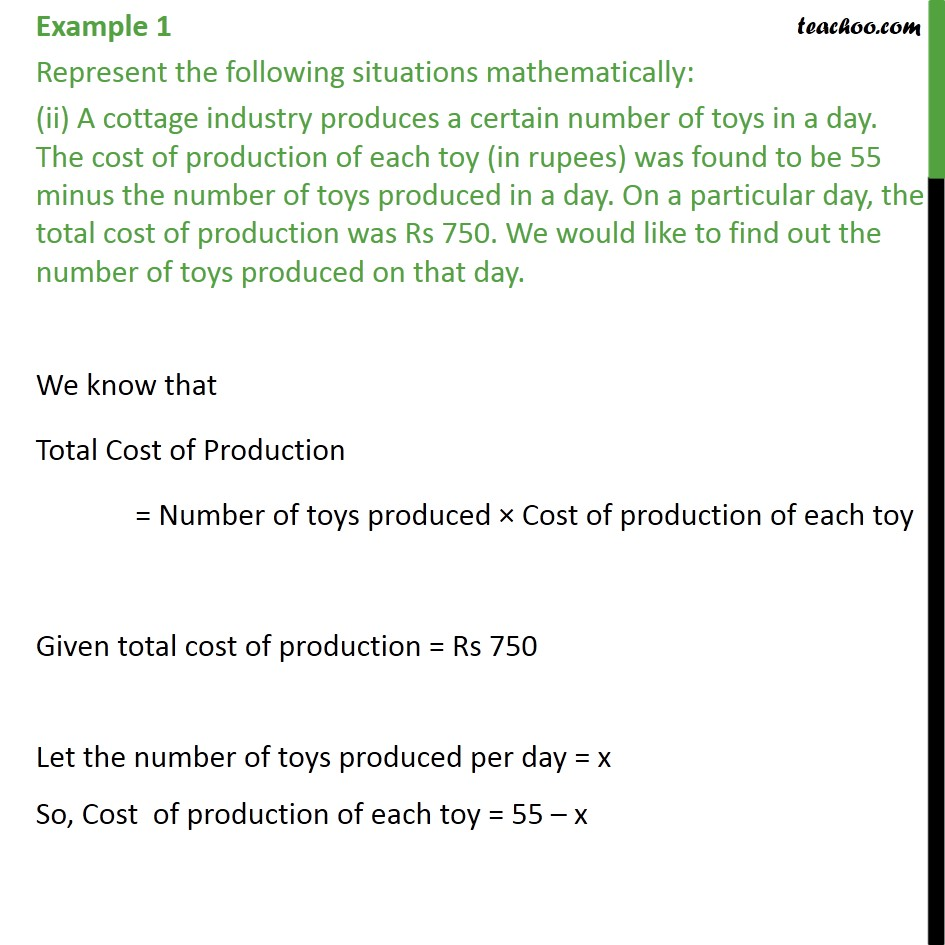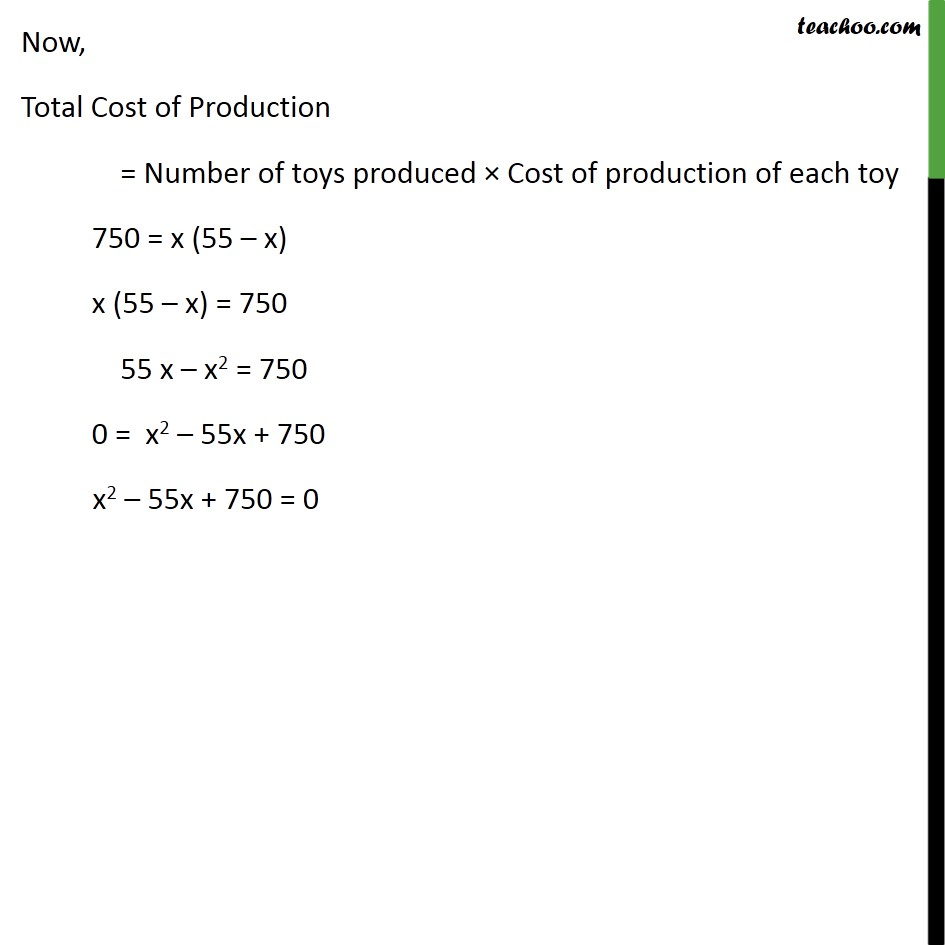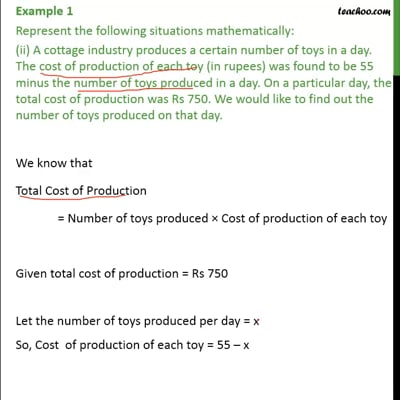Examples

Chapter 4 Class 10 Quadratic Equations
Serial order wiseThis video is only available for Teachoo black users

Learn in your speed, with individual attention - Teachoo Maths 1-on-1 Class

### Transcript

Example 1 Represent the following situations mathematically: (ii) A cottage industry produces a certain number of toys in a day. The cost of production of each toy (in rupees) was found to be 55 minus the number of toys produced in a day. On a particular day, the total cost of production was Rs 750. We would like to find out the number of toys produced on that day. We know that Total Cost of Production = Number of toys produced × Cost of production of each toy Given total cost of production = Rs 750 Let the number of toys produced per day = x So, Cost of production of each toy = 55 – x Now, Total Cost of Production = Number of toys produced × Cost of production of each toy 750 = x (55 – x) x (55 – x) = 750 55 x – x2 = 750 0 = x2 – 55x + 750 x2 – 55x + 750 = 0